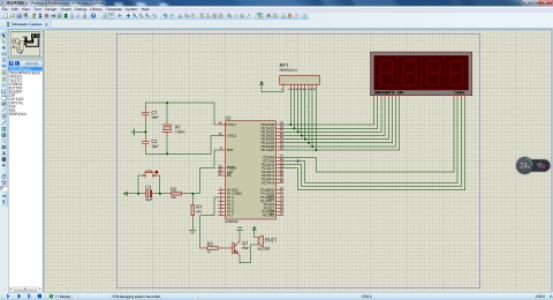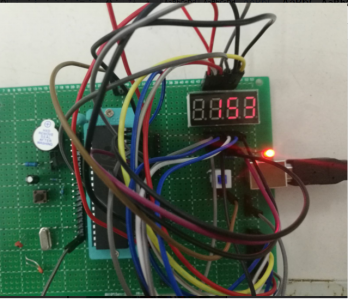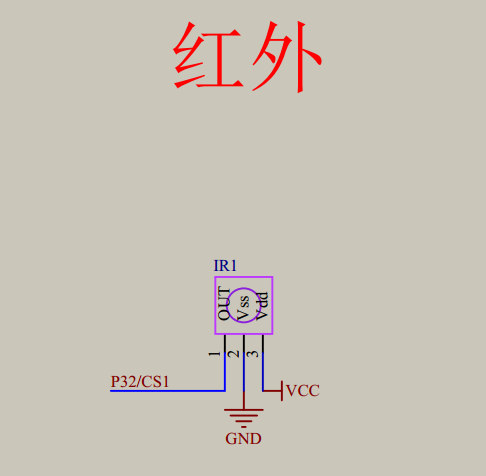2018-05-30 20:20:46 junkaiya 阅读数 4936
• ###### LCD1602和12864显示器-第1季第17部分

本课程是《朱有鹏老师单片机完全学习系列课程》第1季第17个课程，主要讲解LCD1602和LCD12864这两种单片机常用LCD显示器的显示原理、以及英文、中文、图片等如何编程显示。本课程对于显示编程具有重要的启蒙作用，是学习各种深入复杂的显示知识的基础。

4086 人正在学习 去看看 朱有鹏

uchar code table[]=
{0x3f,0x06,0x5b,0x4f,
0x66,0x6d,0x7d,0x07,
0x7f,0x6f,0x77,0x7c,
0x39,0x5e,0x79,0x71};

7SEG-MPX4-CC 四个共阴二极管显示器 1234 是阴公共端；```#include<reg52.h>
#define uchar unsigned char;
#define uint unsigned int;

sbit beep=P1^2;
sbit P2_0 = P2^0;//位选数据的十位，即左边第一位数码管
sbit P2_1 = P2^1;//位选数据的个位，即第二位数码管
sbit P2_2 = P2^2;//位选数据的十位，即第三位数码管
sbit P2_3 = P2^3;//位选数据的个位，即最后一位数码管

unsigned char tcount,a=0,kk=0;
unsigned char table1[]={0x00,0x00,0x00,};//加上你想要显示的手机号对应十六进制

/*     延时1ms的延时函数         */
void delay_ms(unsigned int n)
{
unsigned int i=0,j=0;
for(i=0;i<n;i++)
for(j=0;j<123;j++);
}

/*      数码管显示函数           */
void SMG_display()
{
P0=table1[kk];//第1个数码管显示的数字
P2_0 = 0;
delay_ms(2);
P2_0 = 1;

P0=table1[kk+1];//第2个数码管显示的数字
P2_1 = 0;
delay_ms(2);
P2_1 = 1;

P0=table1[kk+2];//第3个数码管显示的数字
P2_2 = 0;
delay_ms(2);
P2_2 = 1;

P0=table1[kk+3];//第4个数码管显示的数字
P2_3 = 0;
delay_ms(2);
P2_3 = 1;
}

/*       定时器初始化            */
void Timer0Init()
{
TMOD=0x01;    							//定时器0中断方式1
TH0=(65536-5000)/256;						//设初值5000us=5ms
TL0=(65536-5000)%256;
EA=1;								//打开总中断开关
ET0=1;							//T0中断允许
TR0=1;								//T0中断开始（启动T0）
}

/*       定时器中断             */
void timer0() interrupt 1 using 0
{
TH0=(65536-5000)/256;    //无自动重装载功能，需要再次设初值
TL0=(65536-5000)%256;
tcount++;                 //中断循环次数，每次进入中断tcount数值加1

SMG_display();

if(tcount==200)						//200*5000us=1s
{
tcount=0;
kk++;
if(kk>10)
{
kk=0;
beep=0;
delay_ms(50);
beep=1;

}
}
}
/*     主函数                 */
void main()
{
Timer0Init();
while(1);
}

```

2018-05-15 17:07:03 GF__marry 阅读数 968
• ###### LCD1602和12864显示器-第1季第17部分

本课程是《朱有鹏老师单片机完全学习系列课程》第1季第17个课程，主要讲解LCD1602和LCD12864这两种单片机常用LCD显示器的显示原理、以及英文、中文、图片等如何编程显示。本课程对于显示编程具有重要的启蒙作用，是学习各种深入复杂的显示知识的基础。

4086 人正在学习 去看看 朱有鹏
#include <reg52.h>
#define uint8 unsigned char
#define uint16 unsigned short int

//数码管段码显示：0~f,不亮
uint8 code LED_Disp[] = {0xC0,0xF9,0xA4,0xB0,    //这一组编码当中不包含小数点
0x99,0x92,0x82,0xF8,
0x80,0x90}; //十个分别显示 0 1 2 3 4 5 6 7 8 9
uint8 code LED_Disp1[] = {0x40,0x79,0x24,0x30,    //这一个数组的编码中包含小数点
0x19,0x12,0x02,0x78,
0x00,0x10}; //十个分别显示 0 1 2 3 4 5 6 7 8 9

int minute  = 0;
int second = 0;
void mDelay(uint16 mtime)
{
for(; mtime > 0; mtime--)
{
uint8 j = 244;
while(--j);

}

void LED_Disp_Seg7()
{

int minute_1;
int minute_2;
int second_1;
int second_2 ;

if(second == 60)
{
minute++;
second = 0;

if(minute == 60)
{
minute = 0;
}
minute_1 = minute/10;
minute_2 = minute%10;
second_1 = second/10;
second_2 = second%10;
P2 = 0x7f;
P0 = LED_Disp[second_2];
mDelay(5);

P2 = 0xbf;
P0 = LED_Disp[second_1];
mDelay(5);

P2 = 0xdf;
P0 = LED_Disp1[minute_2];
mDelay(5);

P2 = 0xef;
P0 = LED_Disp[minute_1];
mDelay(5);

}
void timeDelay(unsigned int  time)
{
while(time--)
{
LED_Disp_Seg7();
}
}

void main()
{
while(1)
{
timeDelay(80);
second++;
}
}

2019-02-06 22:03:07 cax1165 阅读数 469
• ###### LCD1602和12864显示器-第1季第17部分

本课程是《朱有鹏老师单片机完全学习系列课程》第1季第17个课程，主要讲解LCD1602和LCD12864这两种单片机常用LCD显示器的显示原理、以及英文、中文、图片等如何编程显示。本课程对于显示编程具有重要的启蒙作用，是学习各种深入复杂的显示知识的基础。

4086 人正在学习 去看看 朱有鹏

51单片机中断系统的相关内容：https://blog.csdn.net/cax1165/article/details/86633086``````err=***;
while((irin==*)&&(err>0))
{
delay(*);
err--;
}``````

``````#include<reg52.h>
#define uc unsigned char
#define ui unsigned int
sbit LSA=P2^2;
sbit LSB=P2^3;
sbit LSC=P2^4;
sbit irin=P3^2;

uc code x={0x3f,0x06,0x5b,0x4f,0x66,0x6d,0x7d,0x07,
0x7f,0x6f,0x77,0x7c,0x39,0x5e,0x79,0x71,0X76};//0x76表示H，为十六进制的标志符号
uc dig,irve;
uc t;

void delay(ui i)//延时i*10微秒
{
while(i--);
}

void digdisplay()
{
uc i;
for(i=1;i<=3;i++)
{
switch(i)
{
case(1):
LSA=0;LSB=0;LSC=0;break;
case(2):
LSA=1;LSB=0;LSC=0;break;
case(3):
LSA=0;LSB=1;LSC=0;break;
}
P0=dig[i];
delay(100);
P0=0x00;
}
}

void irinit()
{
IT0=1;
EX0=1;
EA=1;
irin=1;
}

void Ir () interrupt 0
{
uc i,j;ui err;t=0;
delay(700);
if(irin==0)
{
err=1000;
while((irin==0)&&(err>0))
{
delay(1);
err--;
}
if(irin==1)
{
err=500;
while((irin==1)&&(err>0))
{
delay(1);
err--;
}
for(i=1;i<=4;i++)
{
for(j=1;j<=8;j++)
{
err=60;
while((irin==0)&&(err>0))
{
delay(1);
err--;
}
err=500;
while((irin==1)&&(err>0))
{
delay(10);
t++;err--;
if(t>30)
{
return;
}
}
irve[i]>>=1;
if(t>8)
{
irve[i]|=0x80;
}
t=0;
}
}
}
if(irve!=irve) return;
}
}

void main()
{
irinit();
while(1)
{
dig=x;
dig=x[irve%16];
dig=x[irve/16];
digdisplay();
}
}``````

2019-04-04 16:39:58 Labghost 阅读数 460
• ###### LCD1602和12864显示器-第1季第17部分

本课程是《朱有鹏老师单片机完全学习系列课程》第1季第17个课程，主要讲解LCD1602和LCD12864这两种单片机常用LCD显示器的显示原理、以及英文、中文、图片等如何编程显示。本课程对于显示编程具有重要的启蒙作用，是学习各种深入复杂的显示知识的基础。

4086 人正在学习 去看看 朱有鹏

# 控制原理

`CT107D`竞赛板中共有4块`74HC537D`锁存器，它们使`P0`成为控制总线，控制LED灯、四相步进电机、继电器、直流马达、蜂鸣器、`LM386`及数码管显示(或8x8LED矩阵)。另外，138编码器及02或非门，用来选择锁存器，即`P27` `P26` `P25`三个`I\O`口，用于选择锁存器。

## 138编码器及02与非门C B A 被拉低管脚 锁存器编号 对应可控元件
H L L `Y4` `U6` LED灯
H L H `Y5` `U9` 继电器、蜂鸣器等
H H L `Y6` `U8` 数码管位选
H H H `Y7` `U7` 数码管段选

`Y0` `Y1` `Y2`管脚是空闲的，所以控制完成后`P27` `P26` `P25`全部拉低即可。

## 控制LED灯`P27` `P26` `P25`设置成`H` `L` `L`，选择`U6`锁存器，以控制LED灯。

## 控制继电器和蜂鸣器`P27` `P26` `P25`设置成`H` `L` `H`，选择`U9`锁存器，以控制继电器和蜂鸣器。

`ULN2003`是一个高电压、高电流的驱动器，其逻辑上相当于非门。

## 数码管显示`P27` `P26` `P25`设置成`H` `H` `L`，选择`U8`锁存器，即数码管位选。
`P27` `P26` `P25`设置成`H` `H` `H`，选择`U7`锁存器，即数码管段选。

# 代码模板

``````#include<reg52.h>

#ifndef u8
#define u8 unsigned char
#endif

sbit L1 = P0^0;
sbit L2 = P0^1;
sbit RELAY = P0^4;
sbit BUZZ = P0^6;

u8 code font={0xc0,0xf9,0xa4,0xb0,0x99,0x92,0x82,0xf8,0x80,0x90,0xff};
//"共阳数码管"的字模
u8 code y4=0x80;y5=0xa0;y6=0xc0;y7=0xe0;
//用于锁存器片选
u8 dis,val1=123,val2=234;
//用于数码管显示
bit relay=1,buzz=1,l1=0,l2=0;

void display();
void delay100us();		//延时

void delay100us()		//@12.000MHz
{
unsigned char i, j;

i = 2;
j = 39;
do
{
while (--j);
} while (--i);
}

void display(){
u8 i;

//将val1,val2的值,调整成想要的格式
dis=0xbf;
dis=font[val1/100];
dis=font[val1/10%10];
dis=font[val1%10];
dis=0xbf;
dis=font[val2/100];
dis=font[val2/10%10];
dis=font[val2%10];

for(i=0;i<8;i++){
//数码管位选
P2&=0x1f;
P0=1<<i;
P2|=y6;
//数码管段选
P2&=0x1f;
P0=dis[i];
P2|=y7;
delay100us();
P0=0xff;	   //消隐
}
//控制继电器和蜂鸣器
P2&=0x1f;
P0=0x00;RELAY=relay;BUZZ=buzz;
P2|=y5;
//控制LED灯
P2&=0x1f;
P0=0xff;L1=l1;L2=l2;
P2|=y4;
//将P27 P26 P25管脚拉低
P2&=0x1f;
}
int main(){
while(1){
display();
}
}
``````

2013-02-01 15:57:44 jzj1993 阅读数 3763
• ###### LCD1602和12864显示器-第1季第17部分

本课程是《朱有鹏老师单片机完全学习系列课程》第1季第17个课程，主要讲解LCD1602和LCD12864这两种单片机常用LCD显示器的显示原理、以及英文、中文、图片等如何编程显示。本课程对于显示编程具有重要的启蒙作用，是学习各种深入复杂的显示知识的基础。

4086 人正在学习 去看看 朱有鹏

```void main()
{
WELA=1;//1
P1=0xFE;//2
WELA=0;//3

DULA=1;//4
P1=0x06;//5
DULA=0;//6
}``````void main()
{
while(1) {
WELA=1;        //1
P1=0xFE;        //2
WELA=0;        //3
DULA=1;        //4
P1=0x06;        //5
DULA=0;        //6
}
}``````void main()
{
DULA=1;        //1
P1=0x06;        //2
DULA=0;        //3

P1=0xFF;        //4

WELA=1;        //5
P1=0xFE;        //6
WELA=0;        //7
}``````void main()
{
WELA=1;        //1
P1=0xFE;        //2
WELA=0;        //3

DULA=1;        //4
P1=0x06;        //5
DULA=0;        //6
while(1);        //7
}
```

```void main()
{
P1=0xFE;        //1
WELA=1;        //2
WELA=0;        //3

P1=0x06;        //4
DULA=1;        //5
DULA=0;        //6
while(1);        //7
}```

573的 LE锁存使能引脚 高电平时输出和输入相同，低电平时 输出不再改变，即下降沿锁存，也就是低电平锁存。